Bayesian ANOVA: Powerful inference with within-group sample size of 1

By | March 9, 2017

This post is inspired by a question by Dylan Craven that he raised during my Bayesian stats course.

1 Objective

My aim here is to demonstrate that, in Bayesian setting, one can make powerful inference about within-group ANOVA means $\mu_i$ even for groups with sample size $N=1$, i.e. groups with only a single measurement. The main reason this is possible is the assumption of constant variance ($\sigma^2$) among groups: groups with sufficiently large $N$ increase credibility of the $\sigma^2$ estimate, which limits the credible interval of $\mu$ in the group with $N=1$. Conversely, when the assumption is relaxed and $\sigma^2$ is allowed to vary, groups with sample size of 1 are no longer that useful.

2 The data

I will use modified artificially-generated data from the example from Marc Kery’s Introduction to WinBUGS for Ecologists, page 119 (Chapter 9 - ANOVA). The (artificial) data are supposed to describe snout-vent lengths in five populations of Smooth snake (Coronella austriaca); I modified the data so that the first population is only represented by a single observation, as indicated by the arrow in the figure below.

snakes <- read.csv("http://www.petrkeil.com/wp-content/uploads/2017/02/snakes_lengths.csv")

Plotting the data:

par(mfrow=c(1,2), mai=c(0.8,0.8, 0.1, 0.1))
plot(snout.vent ~ population, data=snakes, ylab="Length [cm]")
arrows(x1=1.2, y1=58, x0=2, y0=61, col="red", lwd=2, angle=25, length=0.2)
boxplot(snout.vent ~ population, data=snakes, ylab="Length [cm]", xlab="population", col="grey")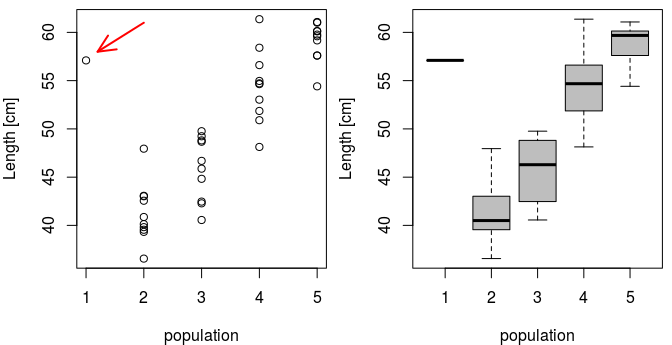3 Fixed-effects ANOVA in JAGS

First, I will model the data using a traditional fixed-effect ANOVA model. For a given snake $i$ in population $j$ the model can be written as:

Where $\mu_j$ is a mean of $j$-th population ($j \in \{1,2,3,4,5\}$), and $i$ identifies individual measurements.

I am interested in a simple question: Is there a statistically significant difference between population 1 and 2? Will I be able to infere the difference even when population 1 has a sample size of 1?

I will fit the model using MCMC in JAGS. Hence, I will prepare the data in a list format:

snake.data <- list(y=snakes$snout.vent, x=snakes$population,
N=nrow(snakes),
N.pop=5)

library(R2jags)

JAGS Model definition:

cat("
model
{
# priors
sigma ~ dunif(0,100) # (you may want to use a more proper gamma prior)
tau <- 1/(sigma*sigma)
for(j in 1:N.pop)
{
mu[j] ~ dnorm(0, 0.0001)
}

# likelihood
for(i in 1:N)
{
y[i] ~ dnorm(mu[x[i]], tau)
}

# the difference between populations 1 and 2:
delta12 <- mu - mu
}
", file="fixed_anova.txt")

And here I fit the model:

model.fit.1 <- jags(data = snake.data,
model.file = "fixed_anova.txt",
parameters.to.save = c("mu", "delta12"),
n.chains = 3,
n.iter = 20000,
n.burnin = 10000,
DIC = FALSE)
## Compiling model graph
##    Resolving undeclared variables
##    Allocating nodes
## Graph information:
##    Observed stochastic nodes: 41
##    Unobserved stochastic nodes: 6
##    Total graph size: 107
##
## Initializing model

Plotting parameter estimates with mcmcplots:

library(mcmcplots)

plot(snakes$snout.vent ~ snakes$population, ylim=c(35, 65), col="grey",
xlab="Population", ylab="Body length")

caterplot(model.fit.1, parms="mu", horizontal=FALSE,
reorder=FALSE, add=TRUE, labels=FALSE, cex=2, col="red")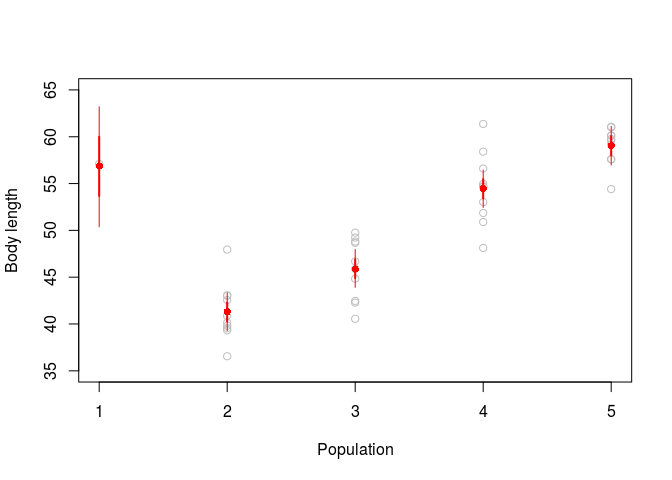The red dots and bars show posterior densities of $\mu$. Grey dots are the data.

So what is the difference between population 1 and 2?

denplot(model.fit.1, parms="delta12", style="plain", mar=c(5,5,2,1), main="",
xlab="Difference between means of populations 1 and 2",
ylab="Posterior density")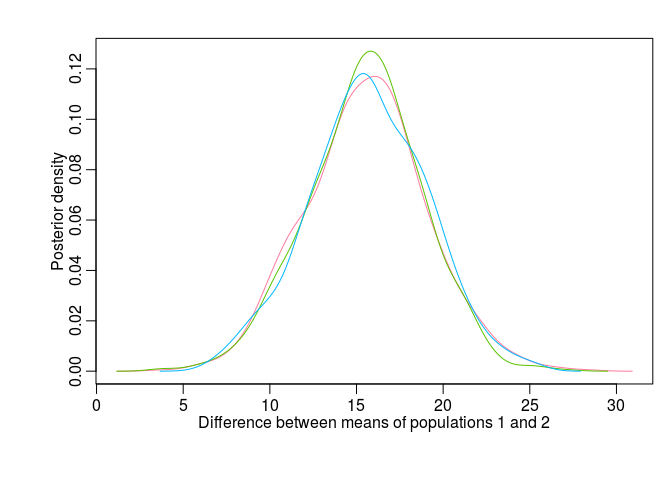There is a clear non-zero difference $\mu_1 - \mu_2$ (the posterior density does not overlap zero). So we can conclude that there is a statistically significant difference between populations 1 and 2, and we can conclude this despite having only $N=1$ for population 1.

4 Relaxing the assumption of constant variance

We can have a look what happens when the assumption of constant $\sigma$ is relaxed. In other words, now every population $j$ has its own $\sigma$, and the model is:

Here is JAGS definition of such model:

cat("
model
{
# priors
for(j in 1:N.pop)
{
mu[j] ~ dnorm(0, 0.0001)T(0,) # Note that I truncate the priors here
sigma[j] ~ dunif(0,100) # again, you may want to use proper gamma prior here
tau[j] <- 1/(sigma[j]*sigma[j])
}

# likelihood
for(i in 1:N)
{
y[i] ~ dnorm(mu[x[i]], tau[x[i]])
}

# the difference between populations 1 and 2:
delta12 <- mu - mu
}
", file="fixed_anova_relaxed.txt")

Let’s fit the model:

model.fit.2 <- jags(data = snake.data,
model.file = "fixed_anova_relaxed.txt",
parameters.to.save = c("mu", "delta12"),
n.chains = 3,
n.iter = 20000,
n.burnin = 10000,
DIC = FALSE)
## Compiling model graph
##    Resolving undeclared variables
##    Allocating nodes
## Graph information:
##    Observed stochastic nodes: 41
##    Unobserved stochastic nodes: 10
##    Total graph size: 140
##
## Initializing model

Here are parameter estimates plotted with mcmcplots

library(mcmcplots)

plot(snakes$snout.vent ~ snakes$population, ylim=c(0, 100), col="grey",
xlab="Population", ylab="Body length")

labels=FALSE, cex=2, col="red")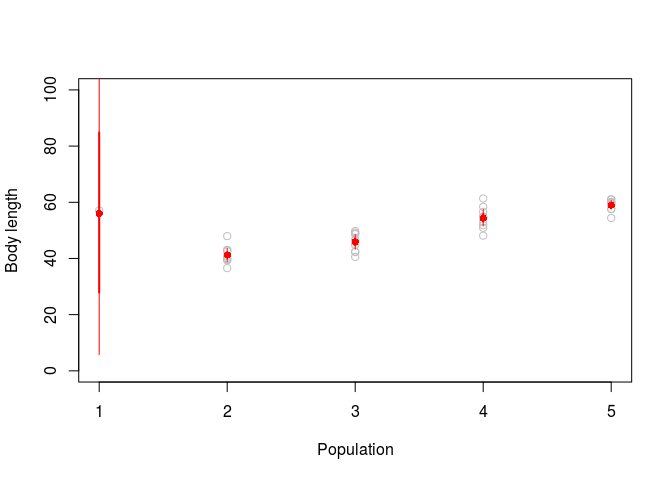Clearly, the posterior density of $\mu_1$ is essentially the priror. In other words, the single observation has little power on its own to overdrive the prior.

We can plot the posterior density of the difference $\mu_1 - \mu_2$

denplot(model.fit.2, parms="delta12", style="plain", mar=c(5,5,2,1), main="",
xlab="Difference between means of populations 1 and 2",
ylab="Posterior density")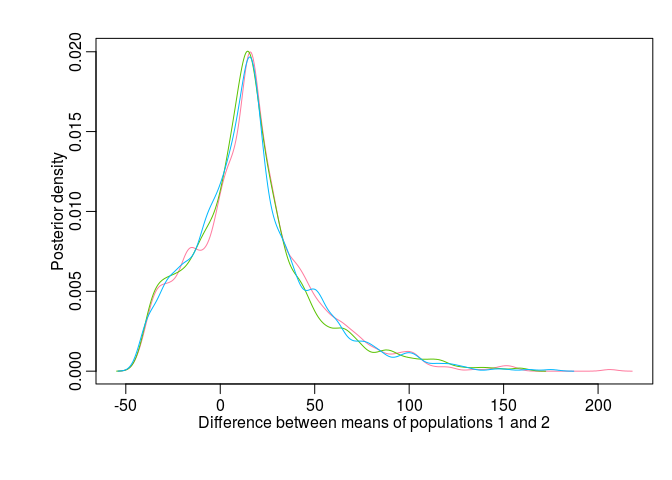And we see that it is all over the place. Huge uncertainty, no clear inference is possible.

5 Conclusion

I have shown that one can make powerful inference about population means even if the sample size for the population is super small (e.g. 1). This is possible when all of the following conditions are met:

1. Bayesian approach to ANOVA must be used.
2. We must have sufficient data from other populations.
3. We must be confident that the assumption of constant variance among the groups is justified.

(Visited 1,758 times, 4 visits today)

4 thoughts on “Bayesian ANOVA: Powerful inference with within-group sample size of 1”

1.Falko

Hi Petr,

Perhaps you can help me as a Bayesian novice: how reliable is Bayesian inference for very large datasets?

For traditional anova, groups with large sample sizes tend to always be significantly different even when these differences are very small (because the degrees of freedom are so high). In these instances, inference is not very helpful and we have to fall back on subjective comparisons of whether an effect size is practically, as opposed to statistically, different. Does Bayesian anova tend to be like this?

In other words, how sensitive is the posterior distribution of the difference between means to sample size? Does it also become more narrowly distributed when sample sizes are very large?

1.Petr Keil Post author

Hi Falko,

To clarify that upfront: effect size and statistical significance (whatever you understand by that) are different things, and should ideally both be reported -- I don't think anyone sane reports just the significance, or just the difference.

Concerning large sample sizes in general: Neither in frequentist nor in Bayesian approach there is a problem with having small but statistically significant difference between groups (detectable thanks to your large sample size) -- it is just not very useful (or interesting), as you mention. Actually, I don't really see any disadvantages of having large datasets, not in Bayesian nor in frequentist approaches either (unless there is a systematic measurement bias that amplifies with more data).

Finally: Yes, posterior distributions of the means will get narrower with increasing sample size. I'd argue that this is a good thing, the more credibility the better. In this respect, it is good to be aware that having narrow posteriors of the means is a completely different thing from the width of your prediction intervals (given sample size and by the variance parameter sigma^2). In other words, you can have highly significant (but small) difference between groups, but when you simulate (predict) from you model, the predictions are still widely spread and all over the place.

Petr

2.Martin Weiser

Hi Petr!

Oh come on, if just the means are not the same among groups, but variances (and other parameters) are (and there is enough data to estimate the within group variance elsewhere - if all populations are of size one, you are doomed, of course), you do not need to simulate and sample at all - this is why we call these "parametric tests" in the frequentist world. 🙂
With good command of calculus, you can profile the probability of overlaps yourself, others (like me) just "look into the tables" (for the simple case). That was a low hanging fruit.
Challenge: with more than one point you can aim for estimating differences in, let us say, within population kurtosis (e.g. stabilizing vs. disruptive selection) - for suchlike problems, the tools in the frequentists' drawer are not that sharp (or that well known in general), while (I suppose) it may be relatively straightforward to do it under Bayesian framework.
Bonus/malus point for the frequentist approach in the case from the blog: you do not get the shrinkage because of the (wannabe uninformative, yet still present) prior.

 summary(lm(snout.vent~as.factor(population),data=snakes))

 Call: lm(formula = snout.vent ~ as.factor(population), data = snakes) Residuals: Min 1Q Median 3Q Max -6.334 -1.466 0.176 1.784 6.906 Coefficients: Estimate Std. Error t value Pr(>|t|) (Intercept) 57.100 3.114 18.338 < 2e-16 *** as.factor(population)2 -15.814 3.266 -4.842 2.43e-05 *** as.factor(population)3 -11.180 3.266 -3.423 0.00156 ** as.factor(population)4 -2.636 3.266 -0.807 0.42488 as.factor(population)5 1.949 3.266 0.597 0.55438 --- Signif. codes: 0 ‘***’ 0.001 ‘**’ 0.01 ‘*’ 0.05 ‘.’ 0.1 ‘ ’ 1 

Residual standard error: 3.114 on 36 degrees of freedom Multiple R-squared: 0.8507, Adjusted R-squared: 0.8341 F-statistic: 51.29 on 4 and 36 DF, p-value: 2.208e-14 

1.Petr Keil Post author

Hi Martin, fair point! I am not saying that it isn't straightforward. However, you actually do see people throwing away data for groups with small samples, believing that such groups are useless. That was also the motivation for writing this post: A dataset isn't necessarily crap just because for some factor levels there is only one, or two, observations. Petr